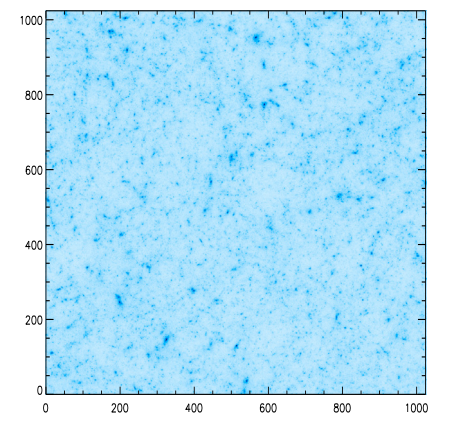# Cosmological model discrimination with weak lensingAuthors: S. Pires, J.-L. Starck, A. Amara, A. Réfrégier, R. Teyssier Journal: Astronomy & Astrophysics Year: 2009 Download: ADS

## Abstract

Weak gravitational lensing provides a unique way of mapping directly the dark matter in the Universe. The majority of lensing analyses use the two-point statistics of the cosmic shear field to constrain the cosmological model, a method that is affected by degeneracies, such as that between σ8 and Ωm which are respectively the rms of the mass fluctuations on a scale of 8 Mpc/h and the matter density parameter, both at z = 0. However, the two-point statistics only measure the Gaussian properties of the field, and the weak lensing field is non-Gaussian. It has been shown that the estimation of non-Gaussian statistics for weak lensing data can improve the constraints on cosmological parameters. In this paper, we systematically compare a wide range of non-Gaussian estimators to determine which one provides tighter constraints on the cosmological parameters. These statistical methods include skewness, kurtosis, and the higher criticism test, in several sparse representations such as wavelet and curvelet; as well as the bispectrum, peak counting, and a newly introduced statistic called wavelet peak counting (WPC). Comparisons based on sparse representations indicate that the wavelet transform is the most sensitive to non-Gaussian cosmological structures. It also appears that the most helpful statistic for non-Gaussian characterization in weak lensing mass maps is the WPC. Finally, we show that the σ8 - Ωmdegeneracy could be even better broken if the WPC estimation is performed on weak lensing mass maps filtered by the wavelet method, MRLens.